# all one question 3. For the following problems, you will be given either the molecular formula of the compound...

all one question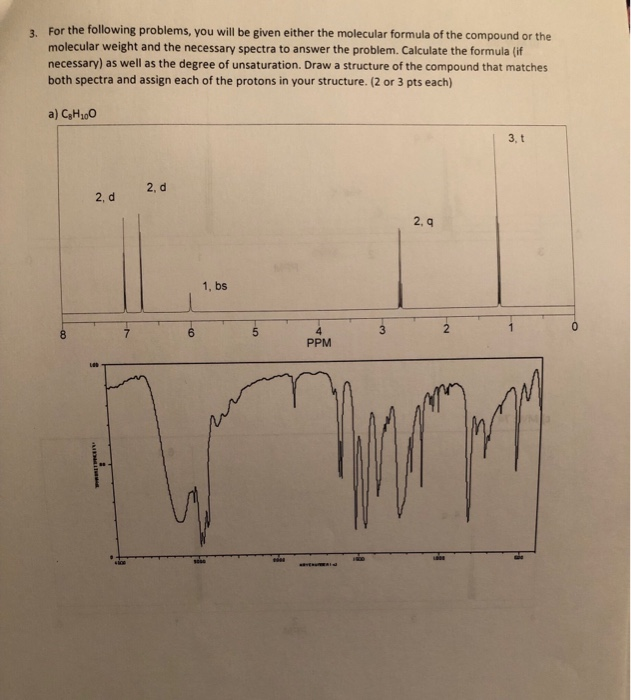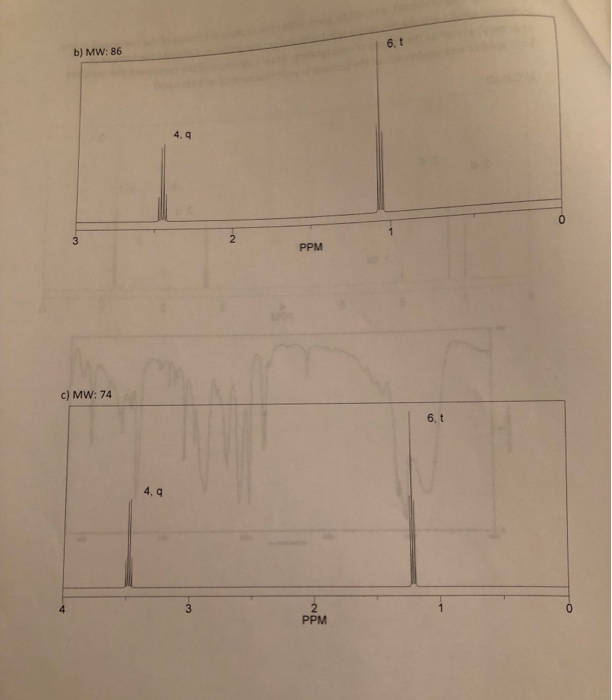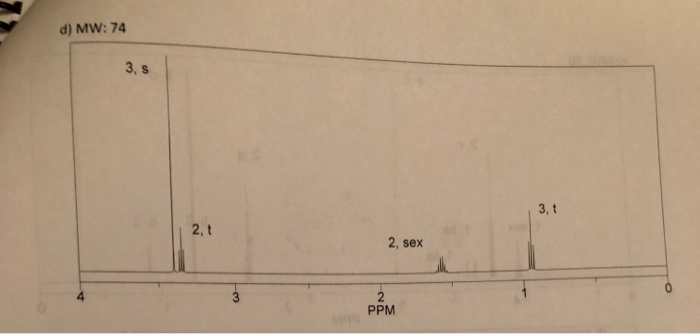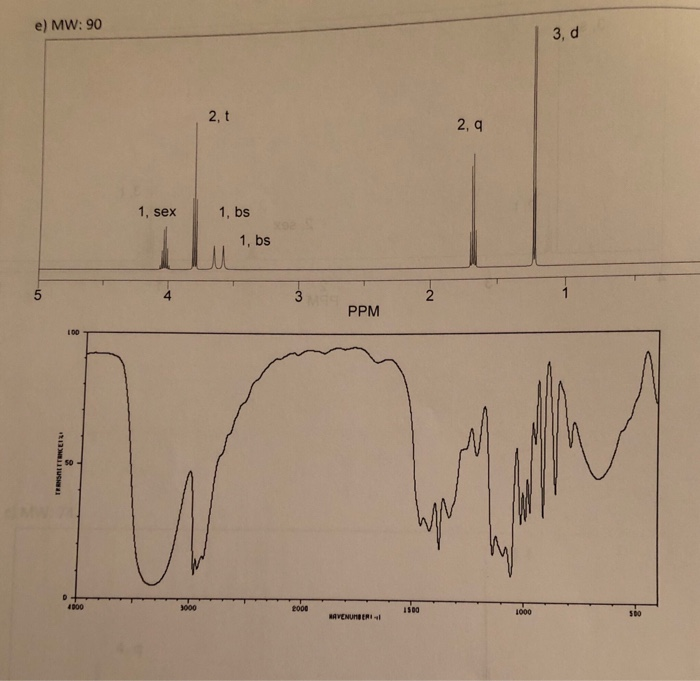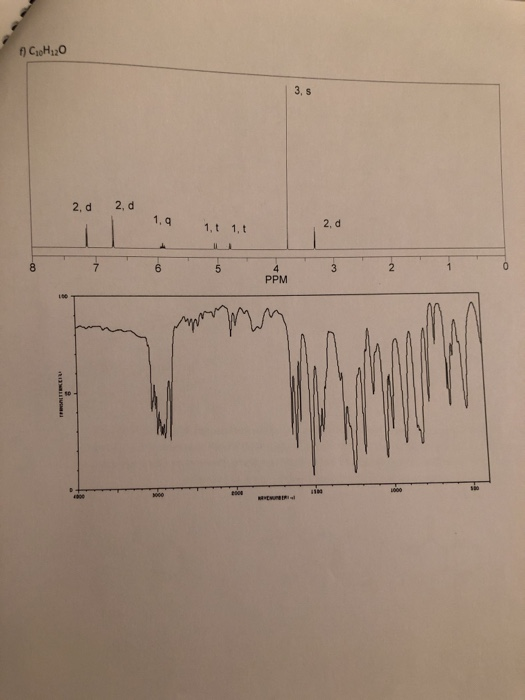3. For the following problems, you will be given either the molecular formula of the compound or the molecular weight and the necessary spectra to answer the problem. Calculate the formula (if necessary) as well as the degree of unsaturation. Draw a structure of the compound that matches both spectra and assign each of the protons in your structure. (2 or 3 pts each) a) C H100 3, t 2, d 2, d 2.q 1, bs 2 4 PPM 6 8 7 -co
6, t b) MW: 86 4, q 2 3 PPM c) MW: 74 6, t 4, q 2 PPM 1 3 C -2
d) MW: 74 3, s 3, t 2, t 2, sex 2 PPM
e) MW: 90 3, d 2, t 2, q 1, sex 1, bs 1, bs 1 5 2 4 3 PPM (00 E so 4000 g000 3000 AS00 1000 S00 AVENUNBERI co 13 1uSH
CsoH2O 3, s 2, d 2, d 1, q 2, d 1,t 1, t 8 7 2 5 4 3 PPM sio L000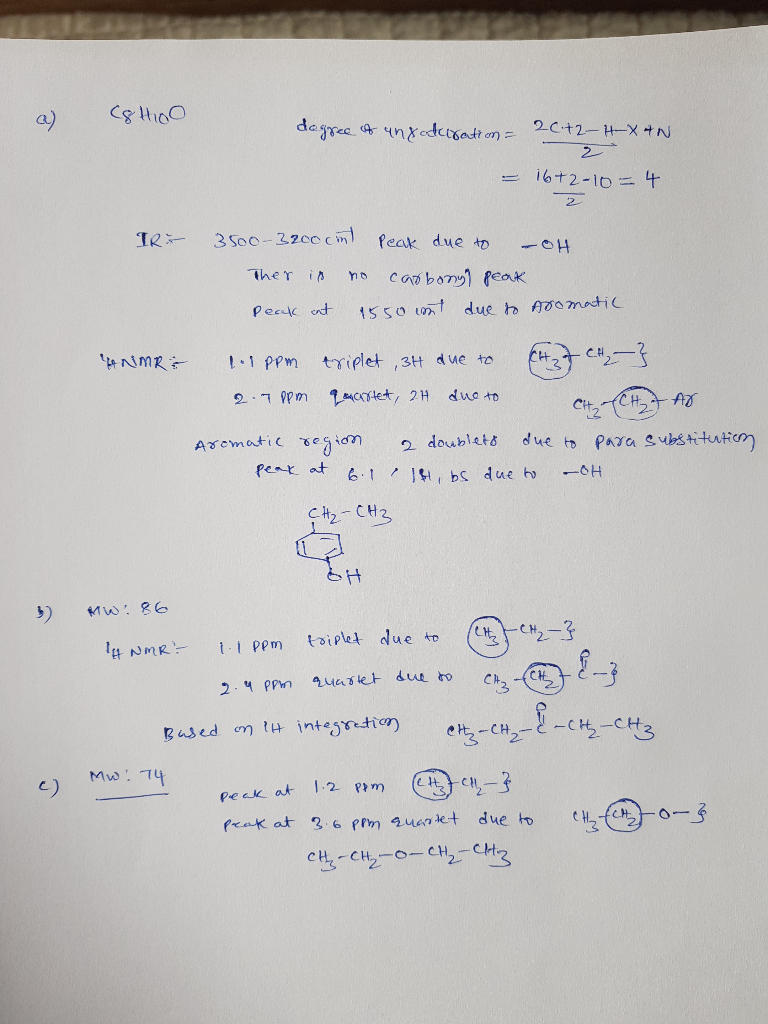##### Add Answer of: all one question 3. For the following problems, you will be given either the molecular formula of the compound...
Similar Homework Help Questions
• ### 5. Identify the following compound based on the molecular formula and given spectra 32. CH, 202 3 total 2 3 Ppm 5. Identify the following compound based on the molecular formula and given sp...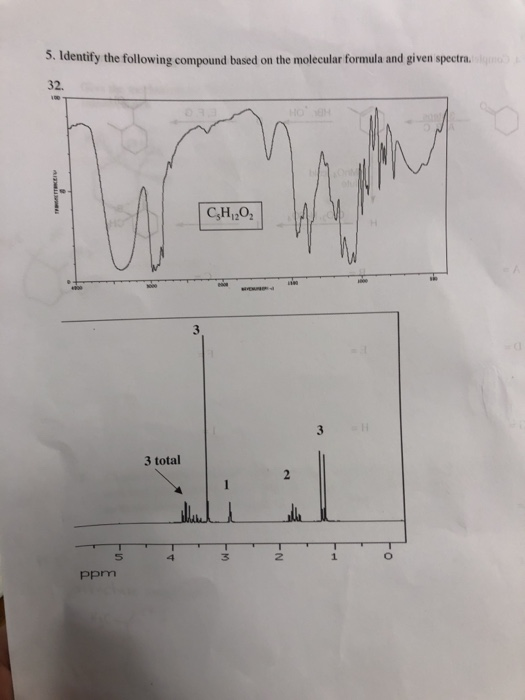5. Identify the following compound based on the molecular formula and given spectra 32. CH, 202 3 total 2 3 Ppm 5. Identify the following compound based on the molecular formula and given spectra 32. CH, 202 3 total 2 3 Ppm

• ### 3) For the following questions draw the structure of the compound based off of the molecular formula and NMR that is gi...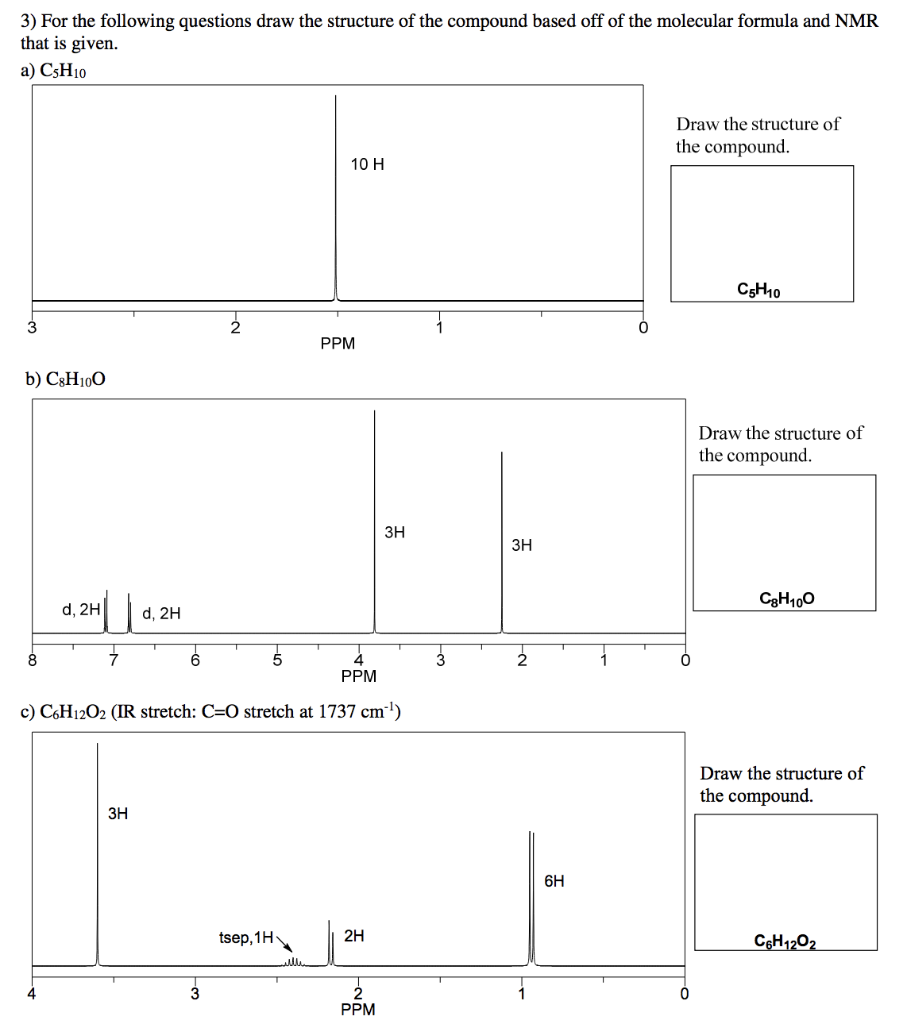3) For the following questions draw the structure of the compound based off of the molecular formula and NMR that is given. a) CsH10 Draw the structure of the compound. 10 H CH10 PPM b) C3H100 Draw the structure of the compound. ЗН ЗН CgH100 d, 240, 2H 6 PPM c) C6H12O2 (IR stretch: C=0 stretch at 1737 cm) Draw the structure of the compound. 6H tsep, 1H C6H1202

• ### Please give the structure based on the NMR spectra depicted. Compound G: Molecular formula = C9H12. Key Infrared signal...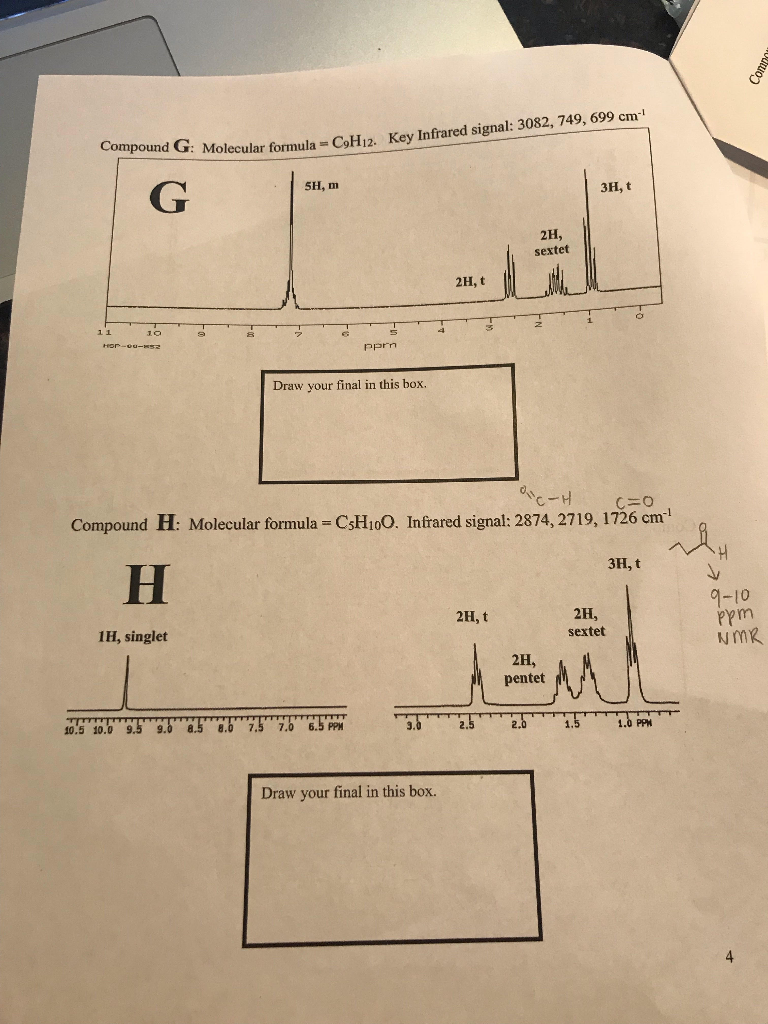Please give the structure based on the NMR spectra depicted. Compound G: Molecular formula = C9H12. Key Infrared signal: 3082, 749, 699 cm 5H, m 3H, t 2H, sextet 2H, t 10 Heoe-ese Pprn Draw your final in this box. Compound H: Molecular formula C\$H10O. Infrared signal: 2874, 2719, 1726 cm 3H, t 9-10 2H, 2H, t udd sextet 1H, singlet 2H, pentet 1.5 2.0 1.0 PPM 3.0 t0 5 10.0 9 9.0 8.5 8.0 7.5 7.0 6.5 PPM Draw...

• ### Please give a structure which follows the following criteria. Compound C 1. Molecular Formula = C12H22...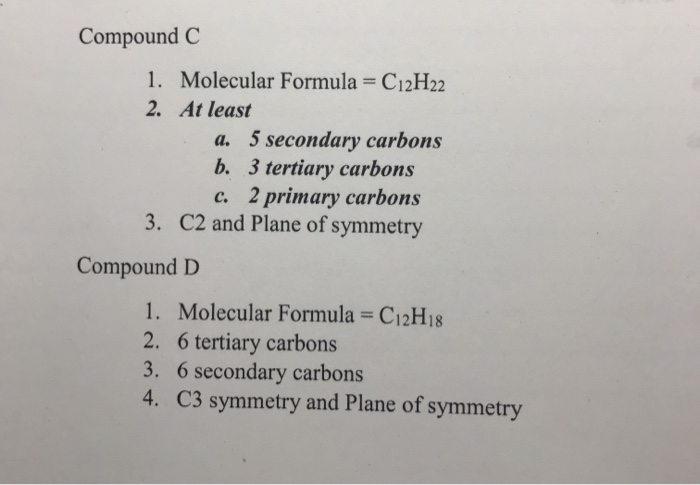Please give a structure which follows the following criteria. Compound C 1. Molecular Formula = C12H22 2. At least a. 5 secondary carbons b. 3 tertiary carbons c. 2 primary carbons 3. C2 and Plane of symmetry Compound D 1. Molecular Formula = C12H18 2. 6 tertiary carbons 3. 6 secondary carbons 4. C3 symmetry and Plane of symmetry Compound C 1. Molecular Formula = C12H22 2. At least a. 5 secondary carbons b. 3 tertiary carbons c. 2 primary...

• ### Which compound gives the following spectra? For the 1H-NMR assign all signals. interpret mol peak and...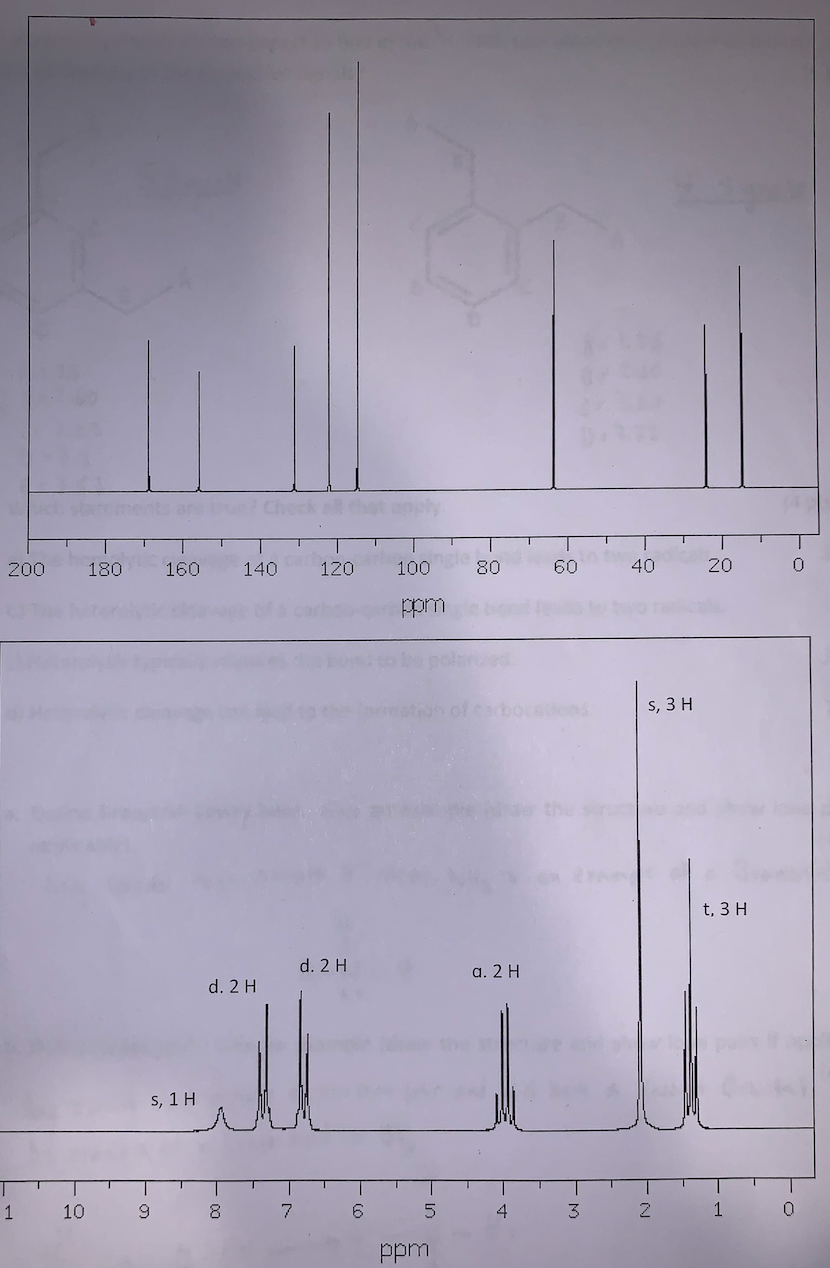Which compound gives the following spectra? For the 1H-NMR assign all signals. interpret mol peak and base peak in the mass spectrum if a mole peak exists. Assign at least two 13C signals. How does the IR spectrum support your finding? Check 200 180 160 120 80 60 40 20 0 140 a 100 ppm s, 3H t, 3 H d. 2 a. 2 H d. 2H s, 1 H 1 10 9 8 7 6 4 3 2 1...

• ### 6. Spectroscopy. The molecular formula of an unknown organic compound is C8H1402. The IR, 1C-NMR and 'H-NMR spectra...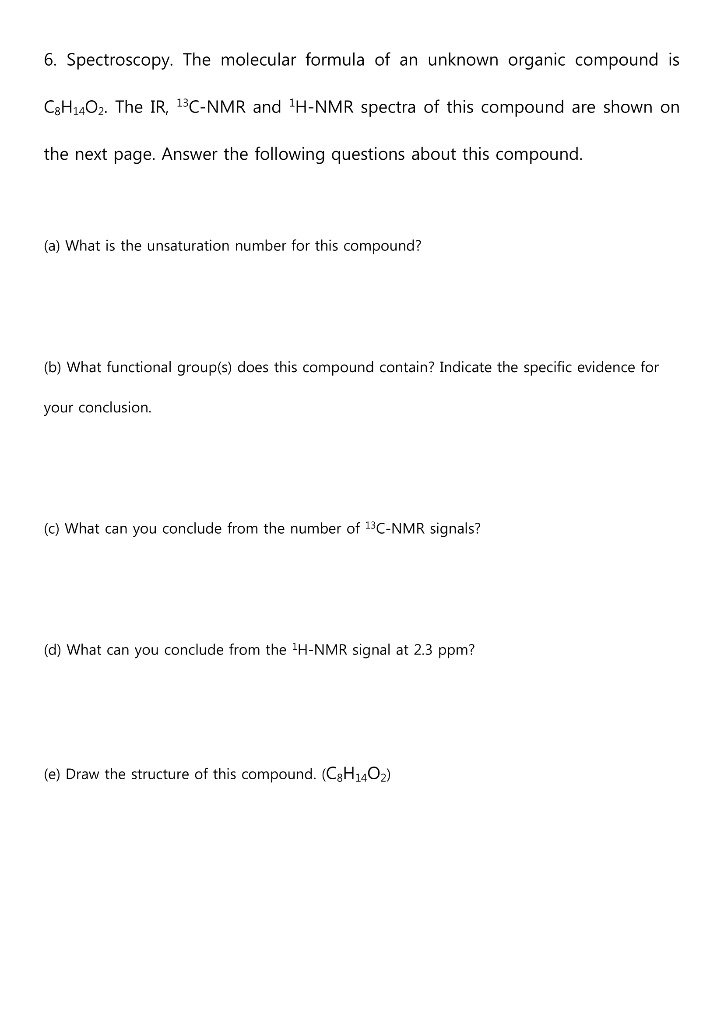6. Spectroscopy. The molecular formula of an unknown organic compound is C8H1402. The IR, 1C-NMR and 'H-NMR spectra of this compound are shown on the next page. Answer the following questions about this compound. (a) What is the unsaturation number for this compound? (b) What functional group(s) does this compound contain? Indicate the specific evidence for your conclusion. (c) What can you conclude from the number of 13C-NMR signals? (d) What can you conclude from the H-NMR signal at 2.3...

• ### 28. A compound with the molecular formula of C3H.ON shows 1H NMR and 13C NMR as...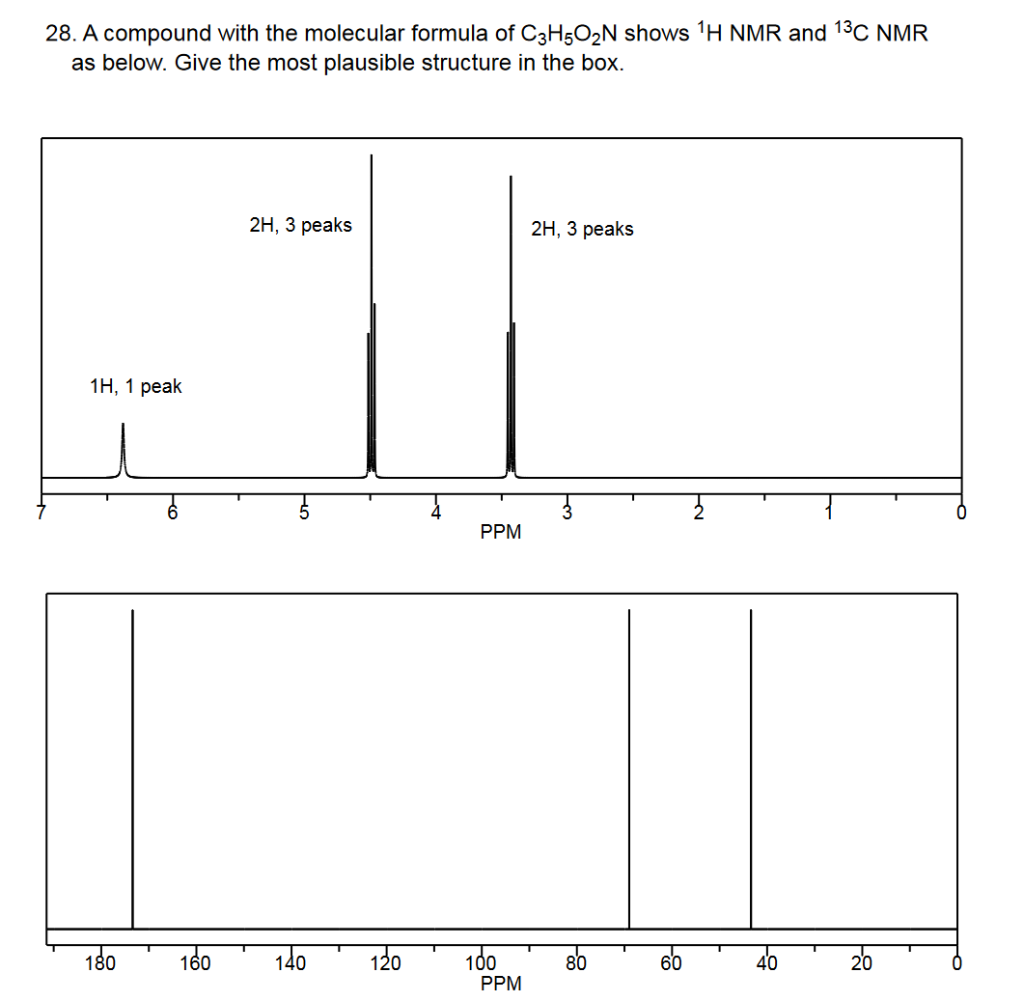28. A compound with the molecular formula of C3H.ON shows 1H NMR and 13C NMR as below. Give the most plausible structure in the box. 2H, 3 peaks 2H, 3 peaks 1H, 1 peak PPM 180 160 140 120 100 80 60 40 20 PPM

• ### You are provided with the molecular formula for each compound. calculate the unsaturation index f...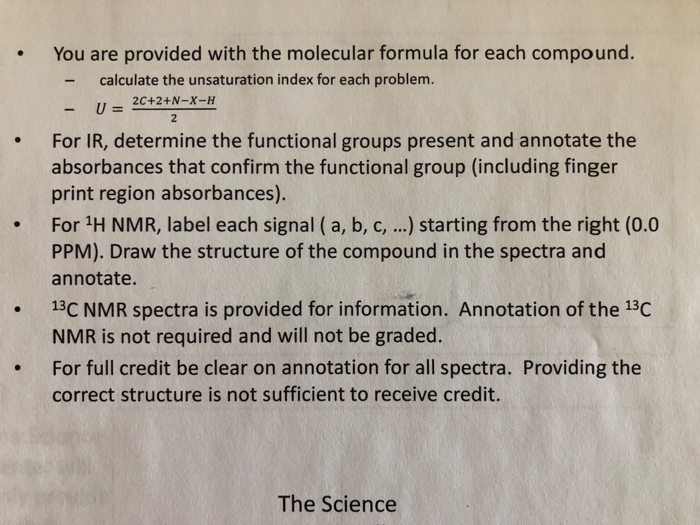You are provided with the molecular formula for each compound. calculate the unsaturation index for each problem. -u2C+2+N-X-H For IR, determine the functional groups present and annotate the absorbances that confirm the functional group (including finger print region absorbances). 2 . For 1H NMR, label each signal (a, b, c,.... starting from the right (0.0 PPM). Draw the structure of the compound in the spectra and annotate. 13C NMR spectra is provided for information. Annotation of the 13C NMR is...

• ### Determine the structure of the compound with molecular formula C7H140 that gives the following spectrum: 21...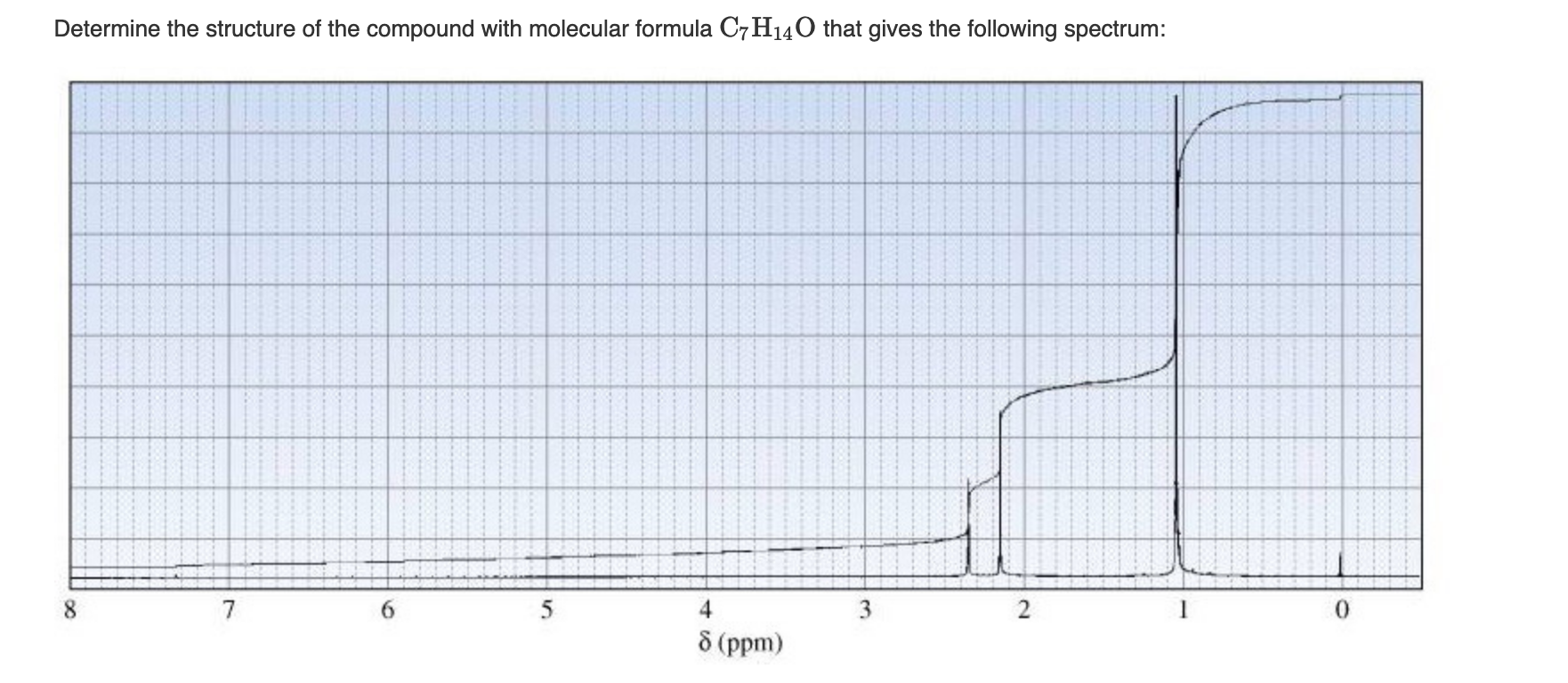Determine the structure of the compound with molecular formula C7H140 that gives the following spectrum: 21 & (ppm)

• ### A compound with molecular formula C7H160 displays the following IR and 'H-NMR spectra. The 13C NMR data suggest that th...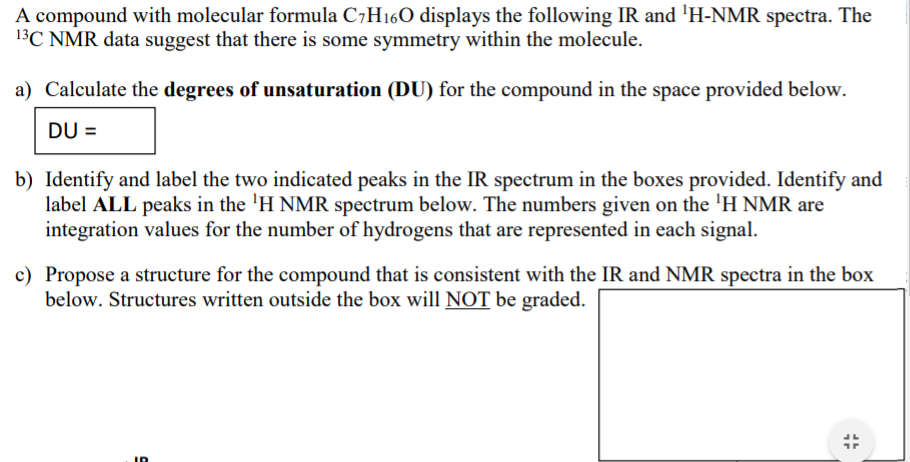A compound with molecular formula C7H160 displays the following IR and 'H-NMR spectra. The 13C NMR data suggest that there is some symmetry within the molecule. a) Calculate the degrees of unsaturation (DU) for the compound in the space provided below. DU = b) Identify and label the two indicated peaks in the IR spectrum in the boxes provided. Identify and label ALL peaks in the 'H NMR spectrum below. The numbers given on the 'H NMR are integration values...

Need Online Homework Help?